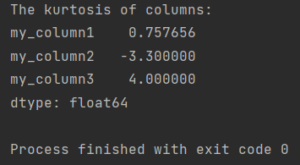# How to calculate kurtosis in Pandas

Here’s how to calculate kurtosis in Pandas..

## How to calculate kurtosis in Pandas

To calculate a Kurtosis in Pandas just use a Kurt method which Pandas is offering to you.

```import pandas as pd

my_df = pd.DataFrame({"my_column1": ['9', '2', '3', '5'],
"my_column2": ['3', '7', '6', '4'],
"my_column3": ['4', '8', '8', '8']})

print(f'The kurtosis of columns:\n{my_df.kurtosis()}')

```
```The kurtosis of columns:
my_column1    0.757656
my_column2   -3.300000
my_column3    4.000000
dtype: float64
```

The kurtosis method calculates the excess kurtosis, which is the kurtosis of a dataset minus the kurtosis of a normal distribution. A positive value of kurtosis indicates that the data has heavier tails than a normal distribution, while a negative value indicates lighter tails.

For more details see the documentation of kurt function.